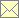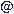Laser Doppler Data Processing Techniques - Bessel's Correction

 Home Scope Literature Data Programs Techniques Links Impressum Data Protection Policy Data Basewebmasternambisde 12.3.2018

For a known true mean value $μ$, the variance of a data set $x i$ can be estimated as

$s 2 = 1 N ∑ i=1 N ( x i - μ ) 2$

If the mean value is not know and the true mean value is replaced by the estimated mean derived from the same data set as

$x ‾ = 1 N ∑ i=1 N x i$

then the variance estimate above will be biased. For independent samples, the estimate

$s 2 = 1 N-1 ∑ i=1 N ( x i - x ‾ ) 2$

is unbiased, where the division by $N-1$ instead of $N$ is widely know as Bessel's correction.

Similar corrections can be made to estimates of the correlation function. Unfortunately, this requires considering that the data samples are correlated — why one would otherwise calculate the correlation function? For equidistantly sampled data, such corrections exist. For irregularly sampled data the numerical effort of the exact correction is not acceptable for practical applications. However, the error of the correlation estimate consists mainly of a constant offset. Therefore, a zero-th order approximation, simply adding an estimate of the estimation variance of the mean estimator to all values of the correlation function, may already lead to acceptable results of the correction. This correction has been adapted to all known estimation principles of correlation functions and power density spectra from randomly sampled LDV data and it is used by default, if the mean value is obtained and subtracted from an LDV data set.

relevant literature: